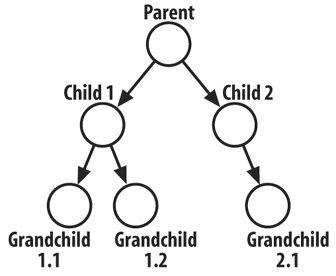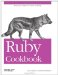# Writing an Iterator Over a Data Structure

Problem

You've created a custom data structure, and you want to implement an each method for it, or you want to implement an unusual way of iterating over an existing data structure.

Solution

Complex data structures are usually constructed out of the basic data structures: hashes, arrays, and so on. All of the basic data structures have defined the each method. If your data structure is composed entirely of scalar values and these simple data structures, you can write a new each method in terms of the each methods of its components.

Here's a simple tree data structure. A tree contains a single value, and a list of children (each of which is a smaller tree).

```	class Tree
def initialize(value)
@value = value
@children = []
end

def <<(value)
subtree = Tree.new(value)
@children << subtree
return subtree
end
end
```

Here's code to create a specific Tree (Figure 7-1):

```	t = Tree.new("Parent")
child1 = t << "Child 1"
child1 << "Grandchild 1.1"
child1 << "Grandchild 1.2"
child2 = t << "Child 2"
child2 << "Grandchild 2.1"
```

Figure 7-1. A simple treeHow can we iterate over this data structure? Since a tree is defined recursively, it makes sense to iterate over it recursively. This implementation of Tree#each yields the value stored in the tree, then iterates over its children (the children are stored in an array, which already supports each) and recursively calls Tree#each on every child tree.

```	class Tree
def each
yield value
@children.each do |child_node|
child_node.each { |e| yield e }
end
end
end
```

The each method traverses the tree in a way that looks right:

```	t.each { |x| puts x }
# Parent
# Child 1
# Grandchild 1.1
# Grandchild 1.2
# Child 2
# Grandchild 2.1
```

Discussion

The simplest way to build an iterator is recursively: to use smaller iterators until you've covered every element in your data structure. But what if those iterators aren't there? More likely, what if they're there but they give you elements in the wrong order? You'll need to go down a level and write some loops.

Loops are somewhat declassé in Ruby because iterators are more idiomatic, but when you're writing an iterator you may have no choice but to use a loop. Here's a reprint of an iterator from Recipe 4.1, which illustrates how to use a while loop to iterate over an array from both sides:

```	class Array
def each_from_both_sides()
front_index = 0
back_index = self.length-1
while front_index <= back_index
yield self[front_index]
front_index += 1
if front_index <= back_index
yield self[back_index]
back_index -= 1
end
end
end
end

%w{Curses! been again! foiled I've}.each_from_both_sides { |x| puts x }
# Curses!
# I've
# been
# foiled
# again!
```

Here are two more simple iterators. The first one yields each element multiple times in a row:

```	module Enumerable
def each_n_times(n)
each { |e| n.times { yield e } }
end
end

%w{Hello Echo}.each_n_times(3) { |x| puts x }
# Hello
# Hello
# Hello
# Echo
# Echo
# Echo
```

The next one returns the elements of an Enumerable in random order; see Recipe 4.10 for a more efficient way to do the shuffling.

```	module Enumerable
def each_randomly
(sort_by { rand }).each { |e| yield e }
end
end
%w{Eat at Joe's}.each_randomly { |x| puts x }
# Eat
# Joe's
# at
```

• Recipe 4.1, "Iterating Over an Array"
• Recipe 4.10, "Shuffling an Array"
• Recipe 5.7, "Iterating Over a Hash"
• Recipe 7.6, " Changing the Way an Object Iterates"
• Recipe 7.8, "Stopping an Iteration"
• Recipe 7.9, "Looping Through Multiple Iterables in Parallel"Ruby Cookbook (Cookbooks (OReilly))
ISBN: 0596523696
EAN: 2147483647
Year: N/A
Pages: 399